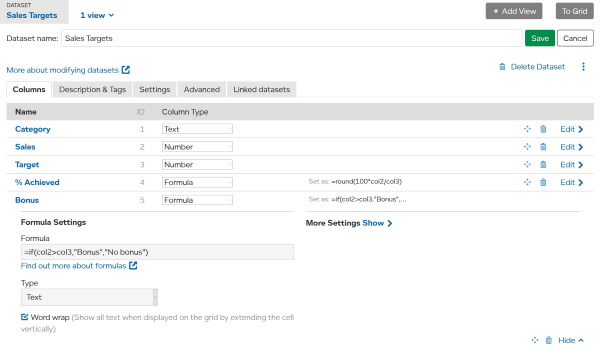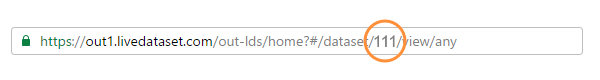## Advanced LiveDataset

Link datasets together to build online systems.

# Using Formulas and Functions

A formula can be used in a calculated column and will be automatically calculated for every row in the dataset.

To enter a formula, the column type must be set to Formula.When a formula refers to a column, use "col" and the column ID number. In the example above the column ID for Sales is 2, so in the formula we use "col2".

A formula can be as simple as

• =100
• ="Hello World"
• = col2 + col3

Formulas can contain numbers, text, and functions including

## Formula Syntax

• A formula always starts with "=".
• Text must be enclosed in double quotes "like this".
• Columns are referenced using "col" and the column ID number.
• Arguments for a formula are encased in round brackets ( ) and separated by commas.

e.g. `=ROUND(col2,1)` will take values from the column that has ID 2 and round them to 1 decimal place.

• The text operator "+" can be used to concatenate text values in columns and text constants

Example: if col1 contains "Live" then the result of formula =col1+"Dataset" is "LiveDataset"

Simple math operators can be used

Operator Description
+ Add
- Subtract
* Multiply
/ Divide
() Use to specify precedence

Precedence examples:

• =100+400/200 following normal mathematical convention, division takes precedence and is calculated first
=100+2 =102

• =(100+400)/200 as ( ) specifies the addition should take precedence it is calculated before dividing by 200
=500/200 =2.5

## Math Functions

Math functions should be used on columns containing numbers.

Function Example
ABS(column)
Absolute values of numbers in the column.
Formula: =ABS(col1)
 col1 result -21.5 21.5 17.31 17.31 44 44
CEILING(column)
Rounds up numbers in the column.
Formula: =CEILING(col1)
 col1 result -21.5 -22 17.31 18 44 44
FLOOR(column)
Rounds down numbers in the column.
Formula: =FLOOR(col1)
 col1 result -21.5 -21 17.31 17 44 44
MAX(column, column)
The maximum value from the columns.
Formula: =MAX(col1,col2)
 col1 col2 result -21.5 79 79 17.31 -8.01 17.31 44 49.45 49.45
MIN(column, column)
The minimum value from the columns.
Formula: =MIN(col1,col2)
 col1 col2 result -21.5 79 -21.5 17.31 -8.01 -8.01 44 49.45 44
ROUND(column)
Rounds numbers in the column to the nearest integer.
Formula: =ROUND(col1)
 col1 result -21.5 -22 17.31 17 44 44
ROUND(column,[places])
Rounds numbers in the column to the number of places specified.
Formula: =ROUND(col1,1)
 col1 result -21.5 -21.5 17.31 17.3 44 44

## Text Functions

Text functions should be used on columns containing text.

Function Example
LEFT(column, length)
The first text characters from text values in the specified column.
length specifies the number of characters.
Formula: =LEFT(col1,4)
 col1 result LiveDataset Live Spreadsheet Spre Database Data
MID(column, start, length)
Returns text characters from the specified column.
start specifies the starting point.
length specifies the number of characters.
Formula: =MID(col1,5,4)
 col1 result LiveDataset Data Spreadsheet adsh Database base
RIGHT(column, length)
The last text characters from the end of text values in the specified column.
length specifies the number of characters.
Formula: =RIGHT(col1,3)
 col1 result LiveDataset set Spreadsheet eet Database ase

## Date Functions

Date functions should be used on columns containing dates.

Function Example
DATEDIF(column, column, value)
The number of days, months or years between dates.
Second column dates are 'subtracted' from first column.
value must be one of:
• "D" for days
• "M" for months
• "Y" for years
Formula: =DATEDIF(col1,col2,"M")
 col1 col2 result 2016-12-31 2017-12-31 -12 2016-02-29 2012-12-31 48 2000-10-29 2000-12-29 -2
DAY(column)
The day of the month as a number.
Formula: =DAY(col1)
 col1 result 2016-12-31 31 2016-02-29 29 2000-10-29 29
DAYADD(column, value or column)
Adds a number of days to a date.
Use negative values to subtract days.
Formula: =DAYADD(col1,col2)
 col1 col2 result 2016-12-31 31 2017-01-31 2016-02-29 0 2016-02-29 2000-10-29 -29 2000-09-30
MONTH(column)
The month for the dates in the column as a number.
Formula: =MONTH(col1)
 col1 result 2016-12-31 12 2016-02-29 2 2000-10-29 10
WEEKDAY(column)
The day of the week for the dates in the column.
result a number from 1 to 7.
Formula: =WEEKDAY(col1)
 col1 result 2016-12-31 7 2016-02-29 2 2000-10-29 1
WEEKNUM(column)
The number of the week for the dates in the column.
result a number from 1 to 53.
Formula: =WEEKNUM(col1)
 col1 result 2016-12-31 53 2016-02-29 10 2000-10-29 45
YEAR(column)
The year for the dates in the column as a number.
Formula: =YEAR(col1)
 col1 result 2016-12-31 2016 2016-02-29 2016 2000-10-29 2000

## Logical and Lookup Functions

.

Function Example
IF(condition, true, false) Tests a condition and returns value from true or false.

condition must include one of:
• == for equals.
• != for not equals.
• > for greater than.
• >= for greater than or equals.
• < for less than.
• <= for less than or equals.
• ISEMPTY(column) to check for empty values.
conditions can be combined using:
• AND to test for 2 conditions to both be true.
• OR to test for at least one of the conditions to be true.
• XOR to test for exactly one condition to be true.
• NOT to test for the condition to NOT be true.
true is the value to return if condition is met.
false is the value to return if condition is not met.

TIP You can compare dates, numbers, and text.
Formula: =IF(col1>100,col1/100,0)
 col1 result 250 2.5 89 0
Formula: =IF(ISEMPTY(col1),"??",col1)
 col1 result 250 250 ?? 89 89
Formula: =IF(col1<100 AND NOT ISEMPTY(col1),"ok","")
 col1 result 250 89 ok
LINK(url) A hyperlink to the url. url can be constructed using other functions. Formula:
=LINK("http://report.com?id="+col1)
 col1 result 250 http://report.com?id=250 http://report.com?id= 89 http://report.com?id=89
VLOOKUP(dataset.column, criteria) Returns values from another dataset where criteria match between the current dataset and the other dataset.

dataset.column is specified as "ds"dataset id."col"column id
e.g. use ds111.col2 for column 2 in dataset 111.

criteria is specified as dataset.column=column to find matching values between a column in the other dataset with a column in the current dataset.

You can include multiple criteria.

TIP Be careful to avoid creating a circular reference.
Other dataset (dataset id=111)
 col1 col2 France €EUR Germany €EUR UK £GBP USA \$USD
Current dataset Formula:
=VLOOKUP(ds111.col2,ds111.col1=col1)
 col1 col2 USA \$USD France €EUR USA \$USD
In this example, dataset 111 holds currencies for countries. The lookup formula matches col1 in the current dataset with col1 in dataset 111 and returns the value of col2 in dataset 111 for that row.

So "USA" in current dataset is looked up in currencies dataset and returns "\$USD".

This is similar to performing a join between database tables.

TIP A quick way to find a dataset id is to look at its url.